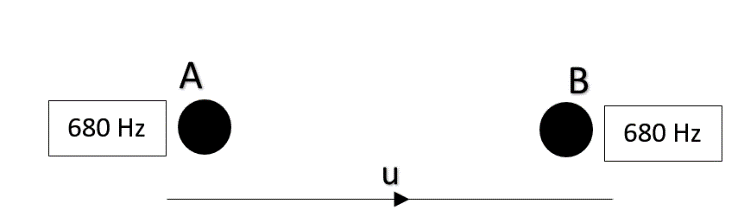QuestionAnswers

# Two sources A and B are sending notes of frequency 680 Hz. An observer starts moving from A to B with a constant velocity ‘u’. If the speed of sound in air is $340\ ms^{-1}$, what must be the value of ‘u’ so that he hears 10 beats per second?$\text{A}. \ 2 ms^{-1}$$\text{B}. \ 2.5 ms^{-1}$$\text{C}. \ 3 ms^{-1}$$\text{D}. \ 3.5 ms^{-1}$Verified
129k+ views
Hint: Beats are the interference pattern between two sources of slightly different frequencies. Beats can only be observed by human ears when the two sources have almost the same frequency. According to Doppler’s effect, when a source emitting some frequency moves with respect to initial position, a change in the frequency is observed, called apparent frequency.

Formula used: $\nu_{apparent}=\nu_{emitted}\left({\dfrac{v_{\circ}+v_{observer}}{v_{\circ}-v_{source}}}\right)$, $Beat\ frequency (\beta)=|\nu_1-\nu_2|$where $\nu_{\circ}$is the speed of sound in air.

Let us first derive the expression in which the two sources are at rest and the observer is moving.The apparent frequency of source ‘A’ will be ($\nu_{A}$):
$\nu_{A} = 680 \times \left( \dfrac{340-u}{340}\right )$ …………①
$\nu_{B} = 680 \times \left( \dfrac{340+u}{340}\right )$ ………….②
Notice in case of ‘A’, the sign is negative because the observer is moving away from the source and hence the apparent frequency will be less and hence the numerator term is greater. Whereas in ‘B’, the sign is positive which is because the observer is moving towards the source and hence the apparent frequency will be more, hence the numerator term must be greater.
Now, to get beats, putting the two frequencies in beat equation, we get:
$\beta=|\nu_1-\nu_2|$
Now as beats is a number, hence must be positive, therefore:
$\beta = \nu_B - \nu_A$
Or $\beta = 680 \times \left( \dfrac{340+u}{340}\right ) -680 \times \left( \dfrac{340-u}{340}\right )$
$\beta=\dfrac{680}{340} (340 + u - 340 +u) = \dfrac{680}{340} \times 2u$
But as per the question, we require 10 beats per second.
Hence $10 = \dfrac{680}{340} \times 2u$
Solving for u, we get:
$u=2.5 ms^{-1}$

So, the correct answer is “Option B”.

Note: In the Doppler effect formula, as the source is at rest, we didn’t alter the denominator term. But if the sources also started moving, we have to apply the logic that if the source is approaching the observer, there must be an increment in frequency. And so we have to decrease the denominator to increase the net term and vice-versa.